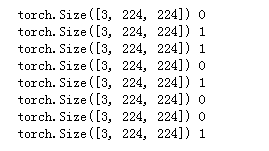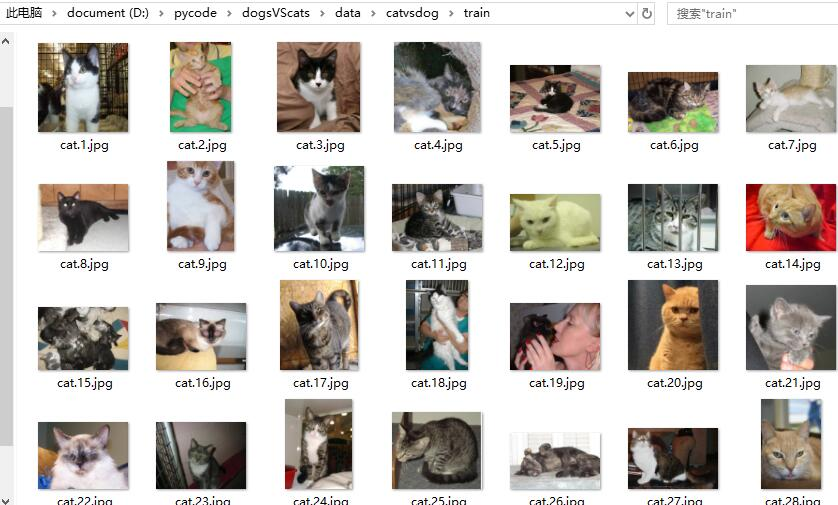2018-12-19 15:34:27 weixin_40123108 阅读数 216
• ###### 深度学习与PyTorch入门实战教程

亚洲排名第1的新加坡国立大学AI团队倾情打造，资深研究员龙龙老师主讲，帮助人工智能、深度学习初学者快速、深刻理解深度学习算法原理与实践。 【莫烦老师】权威推荐：在教学中，龙龙老师以简短高效的方式，从深度学习的多个角度向我们展开了论述，非常适合想对深度学习有全方位了解的朋友。 【PyTorch中文网】：讲解简单易懂、由浅入深，是一门值得推荐的课程。 课程特色： 1. 通俗易懂，快速入门 对深度学习算法追本溯源、循序渐进式讲解，学员不需要任何机器学习基础，只需要写过代码即可轻松上手。 2. 实用主导，简单高效 使用新手最容易掌握的深度学习框架PyTorch实战，比起使用TensorFlow的课程难度降低了约50%，而且PyTorch是业界最灵活，最受好评的框架。 3. 案例为师，实战护航 基于计算机视觉和NLP领域的经典数据集，从零开始结合PyTorch与深度学习算法完成多个案例实战。 4. 持续更新，永久有效 一次购买，永久有效。深度学习课程会持续更新下去，内容和学术界最前沿算法保持同步。

3776 人正在学习 去看看 龙良曲

## 数据加载

getitem：返回一条数据，或一个样本。obj[index]等价于obj.getitem(index)
len：返回样本的数量。len(obj)等价于obj.len()

Kaggle经典挑战赛"Dogs vs. Cat"的数据为例，来详细讲解如何处理数据。"Dogs vs. Cats"是一个分类问题，判断一张图片是狗还是猫，其所有图片都存放在一个文件夹下，根据文件名的前缀判断是狗还是猫。

data/dogcat/
|-- cat.12484.jpg
|-- cat.12485.jpg
|-- cat.12486.jpg
|-- cat.12487.jpg
|-- dog.12496.jpg
|-- dog.12497.jpg
|-- dog.12498.jpg
`-- dog.12499.jpg

``````import torch as t
from torch.utils import data
import os
from PIL import  Image
import numpy as np

class DogCat(data.Dataset):
def __init__(self, root):
imgs = os.listdir(root)
# 所有图片的绝对路径
# 这里不实际加载图片，只是指定路径，当调用__getitem__时才会真正读图片
self.imgs = [os.path.join(root, img) for img in imgs]

def __getitem__(self, index):
img_path = self.imgs[index]
# dog->1， cat->0
label = 1 if 'dog' in img_path.split('/')[-1] else 0
pil_img = Image.open(img_path)
array = np.asarray(pil_img)
data = t.from_numpy(array)
return data, label

def __len__(self):
return len(self.imgs)
``````

``````dataset = DogCat('./data/dogcat/')
img, label = dataset # 相当于调用dataset.__getitem__(0)
for img, label in dataset:
print(img.size(), img.float().mean(), label)
``````

(https://img-blog.csdnimg.cn/20181219152658954.png)

**

• 返回样本的形状不一，因每张图片的大小不一样，这对于需要取batch训练的神经网络来说很不友好
• 返回样本的数值较大，未归一化至[-1, 1]

**

• Scale：调整图片尺寸，长宽比保持不变

• CenterCrop、RandomCrop、RandomSizedCrop： 裁剪图片

• Pad：填充 ToTensor：将PIL Image对象转成Tensor，会自动将[0, 255]归一化至[0, 1]

**Normalize：**标准化，即减均值，除以标准差
**ToPILImage：**将Tensor转为PIL Image对象

``````import os
from PIL import  Image
import numpy as np
from torchvision import transforms as T

transform = T.Compose([
T.Resize(224), # 缩放图片(Image)，保持长宽比不变，最短边为224像素
T.CenterCrop(224), # 从图片中间切出224*224的图片
T.ToTensor(), # 将图片(Image)转成Tensor，归一化至[0, 1]
T.Normalize(mean=[.5, .5, .5], std=[.5, .5, .5]) # 标准化至[-1, 1]，规定均值和标准差
])

class DogCat(data.Dataset):
def __init__(self, root, transforms=None):
imgs = os.listdir(root)
self.imgs = [os.path.join(root, img) for img in imgs]
self.transforms=transforms

def __getitem__(self, index):
img_path = self.imgs[index]
label = 0 if 'dog' in img_path.split('/')[-1] else 1
data = Image.open(img_path)
if self.transforms:
data = self.transforms(data)
return data, label

def __len__(self):
return len(self.imgs)

dataset = DogCat('./data/dogcat/', transforms=transform)
img, label = dataset
for img, label in dataset:
print(img.size(), label)
``````1. torchvision.transforms.Compose(transforms)

transforms： 由transform构成的列表

``````transforms.Compose([
transforms.CenterCrop(224),
transforms.ToTensor(),
])
``````

2

CenterCrop(size)

size可以是tuple，(target_height, target_width)。
size也可以是一个Integer，在这种情况下，切出来的图片的形状是正方形。

4、RandomHorizontalFlip

5、RandomSizedCrop(size, interpolation=2)

7、Normalize(mean, std)

8、ToTensor

9、ToPILImage

10、Lambda(lambd)

11、Scale(size, interpolation=2)

1. pytorch中tensor和numpy的通道位置不同，numpy的通道在H，W之后，即（H，W，C），用np.shape(object)可以查看；而tensor的通道在H和W之前，即（C，H，W），用np.shape(object)或者object.shape可以查看；

1. 处理针对的是tensor格式，需要通过from_numpy等方法，将输入图像时的numpy格式转换为tensor格式

2. 进行Normalize等处理，需要数据类型为float

1.`torchvision.transforms.ToTensor()`

``````from PIL import Image
img1 = Image.open('./1.jpg')

t_out = transforms.ToTensor()(img1)
``````

shape为(H,W,C)的numpy.ndarray，转换成形状为[C,H,W]，取值范围是[0,1.0]的Tensor

``````n_out = np.random.rand(100,100,3)
print(n_out.dtype)

t_out = transforms.ToTensor()(n_out)
print(t_out.type())
``````

float64
torch.DoubleTensor

2.`torchvision.transforms.ToPILImage()`

ndarray的数据类型要求dtype=uint8, range[0, 255] and shape H x W x C
Tensor 的shape为 C x H x W 要求是FloadTensor的，不允许DoubleTensor或者其他类型
# to a PIL.Image of range [0, 255]

#初始化随机数种子

``````np.random.seed(0)

data = np.random.randint(0, 255, 300)
print(data.dtype)
n_out = data.reshape(10,10,3)

#强制类型转换
n_out = n_out.astype(np.uint8)
print(n_out.dtype)

img2 = transforms.ToPILImage()(n_out)
img2.show()
``````

``````t_out = torch.randn(3,10,10)
img1 = transforms.ToPILImage()(t_out)
img1.show()
``````

``````t_out = torch.randn(3,10,10)
img1 = transforms.ToPILImage()(t_out.float())
img1.show()
``````

pytorch处理大数据 相关内容

2019-02-26 21:18:17 qq_33760223 阅读数 120
• ###### 深度学习与PyTorch入门实战教程

亚洲排名第1的新加坡国立大学AI团队倾情打造，资深研究员龙龙老师主讲，帮助人工智能、深度学习初学者快速、深刻理解深度学习算法原理与实践。 【莫烦老师】权威推荐：在教学中，龙龙老师以简短高效的方式，从深度学习的多个角度向我们展开了论述，非常适合想对深度学习有全方位了解的朋友。 【PyTorch中文网】：讲解简单易懂、由浅入深，是一门值得推荐的课程。 课程特色： 1. 通俗易懂，快速入门 对深度学习算法追本溯源、循序渐进式讲解，学员不需要任何机器学习基础，只需要写过代码即可轻松上手。 2. 实用主导，简单高效 使用新手最容易掌握的深度学习框架PyTorch实战，比起使用TensorFlow的课程难度降低了约50%，而且PyTorch是业界最灵活，最受好评的框架。 3. 案例为师，实战护航 基于计算机视觉和NLP领域的经典数据集，从零开始结合PyTorch与深度学习算法完成多个案例实战。 4. 持续更新，永久有效 一次购买，永久有效。深度学习课程会持续更新下去，内容和学术界最前沿算法保持同步。

3776 人正在学习 去看看 龙良曲

在深度学习中，数据的处理对于神经网络的训练来说十分重要，良好的数据（包括图像、文本、语音等）处理不仅可以加速模型的训练，同时也直接关系到模型的效果。本文以处理图像数据为例，记录一些使用PyTorch进行图像预处理和数据加载的方法

### 数据的加载

在PyTorch中，数据加载需要自定义数据集类，并用此类来实例化数据对象，实现自定义的数据集需要继承torch.utils.data包中的Dataset类
在继承Dataset实现自己的类时，需要实现以下两个Python魔法方法：

• __getitem__(index): 返回一个样本数据，当使用obj[index]时实际就是在调用obj.__getitem__(index)

• __len__()：返回样本的数量，当使用len(obj)时实际就是在调用obj.__len__()

例如，以猫狗大战的二分类数据集为例，其加载过程如下：``````import os
import torch as t
from torch.utils import data
from PIL import Image
import numpy as np

class dogCat(data.Dataset):
def __init__(self,root): # root为数据存放目录
imgs = os.listdir(root) #列出当前路径下所有的文件
self.imgs = [os.path.join(root,img) for img in imgs] # 所有图片的路径
#print(self.imgs)

"""返回一个样本数据"""
def __getitem__(self, item):
img_path = self.imgs[item] # 第item张图片的路径
#dog 1 cat 0
label = 1 if 'dog' in img_path.split('\\')[-1] else 0 # 获取标签信息
#print(label)
pil_img = Image.open(img_path) #读入图片
print(type(pil_img))
array = np.asarray(pil_img) # 转为numpy.array类型
data = t.from_numpy(array) # 转为tensor类型
return data,label #返回图片对应的tensor及其标签

"""样本的数量"""
def __len__(self):
return len(self.imgs)

if __name__ == '__main__':
dogcat = dogCat('D:\pycode\dogsVScats\data\catvsdog\\train') #数据集对象
data,label = dogcat # 返回第0张图片的信息
print(data.size())
print(label)
print(len(dogcat))
``````

### 计算机视觉工具包：torchvision

对于图像数据来说，以上的数据加载时不完善的，因为只是将图片读入，而没有进行相关的处理，如每张图片的大小和形状，样本的数值归一化等等。
为了解决这一问题，PyTorch开发了一个视觉工具包torchvision，这个包独立于torch，需要通过`pip install torchvision`来单独安装。
torchvision有三个部分组成：

• models提供各种经典的网络结构和预训练好的模型，如AlexNet、VGG、ResNet、Inception等
``````from torchvision import models
from torch import nn
resnet34 = models.resnet34(pretrained=True,num_classes=1000) # 加载预训练模型
resnet34.fc=nn.Linear(512,10) # 修改全连接层为10分类
``````
• datasets提供了常用的数据集，如MNIST、CIFAR10/100、ImageNet、COCO等
``````from torchvision import datasets
``````

除了常用数据集外，需要特别注意的是ImageFolder，ImageFolder假设所有的文件按文件夹存放，每个文件夹下面存储同一类的图片，文件夹的名字为这一类别的名字。这是我们经常用到的一种数据组织形式。

``````# 使用方法：
# 参数：文件夹路径，对图像做什么样的转换，对标签做什么样的转换，如何加载图片

from torchvision.datasets import ImageFolder
dataset = ImageFolder('data\\')
print(dataset.class_to_idx) # class_to_idx ,label和id的对应关系，从0开始
print(dataset.imgs) # 数据和标签对应
``````
• transforms: 提供常用的数据预处理操作，主要是对Tensor和PIL Image对象的处理操作

对Tensor的操作：Normalize、ToPILImage等。

如果要进行多个操作，可以通过transforms.Compose([])将操作拼接起来。但是需要注意的是需要首先构建转换操作，然后再执行转换操作。

``````import os
from torch.utils import data
from PIL import Image
import numpy as np
from torchvision import transforms as T

transform = T.Compose([T.Resize(224),T.CenterCrop(224),T.ToTensor(),T.Normalize(mean=[.5,.5,.5],std=[.5,.5,.5])])  # 构建转换操作

class dogCat(data.Dataset):
def __init__(self,root,transforms):
imgs = os.listdir(root)
#print(imgs)
self.imgs = [os.path.join(root,img) for img in imgs]
#print(self.imgs)
self.transforms = transforms

def __getitem__(self, item):
img_path = self.imgs[item]
#dog 1 cat 0
label = 1 if 'dog' in img_path.split('\\')[-1] else 0
#print(label)
pil_img = Image.open(img_path)
if self.transforms:
pil_img = self.transforms(pil_img)  #执行准换操作
return pil_img,label,item

def __len__(self):
return len(self.imgs)

``````

通过上述描述，我们通过自定义数据集类，使用视觉工具包进行图像的转换等操作，最终得到的是一个dataset的数据集对象，使用此对象可以一次返回一个样本。

``````DataLoader(dataset,batch_size=1,shuffle=False,sampler=None,num_workers=0,collate_fn=default_collate,pin_memory=False,drop_last=False)
``````

参数含义如下：

• dataset：加载的数据集

• batch_zize: 批大小

• shuffle： 是否将数据打乱

• sampler：样本抽样，常用的有随机采样RandomSampler，shuffle=True时自动调用随机采样，默认是顺序采样，还有一个常用的是：WeightedRandomSampler，按照样本的权重进行采样。

• num_workers: 使用的进程数，0代表不使用多进程。

• collate_fn: 拼接方式。

• pin_memory: 是否将数据保存在pin memory区。

• drop_last: 是否将多出来的不足一个batch的丢弃。

``````from torchvision import transforms as T

transform = T.Compose([T.Resize(224),T.CenterCrop(224),T.ToTensor(),T.Normalize(mean=[.5,.5,.5],std=[.5,.5,.5])])

if __name__ == '__main__':
dogcat = dogCat('D:\pycode\dogsVScats\data\catvsdog\\train', transform)
data, label, index = dogcat

train()
``````

### 总结

pytorch处理大数据 相关内容

2017-07-25 21:05:02 u011276025 阅读数 7398
• ###### 深度学习与PyTorch入门实战教程

亚洲排名第1的新加坡国立大学AI团队倾情打造，资深研究员龙龙老师主讲，帮助人工智能、深度学习初学者快速、深刻理解深度学习算法原理与实践。 【莫烦老师】权威推荐：在教学中，龙龙老师以简短高效的方式，从深度学习的多个角度向我们展开了论述，非常适合想对深度学习有全方位了解的朋友。 【PyTorch中文网】：讲解简单易懂、由浅入深，是一门值得推荐的课程。 课程特色： 1. 通俗易懂，快速入门 对深度学习算法追本溯源、循序渐进式讲解，学员不需要任何机器学习基础，只需要写过代码即可轻松上手。 2. 实用主导，简单高效 使用新手最容易掌握的深度学习框架PyTorch实战，比起使用TensorFlow的课程难度降低了约50%，而且PyTorch是业界最灵活，最受好评的框架。 3. 案例为师，实战护航 基于计算机视觉和NLP领域的经典数据集，从零开始结合PyTorch与深度学习算法完成多个案例实战。 4. 持续更新，永久有效 一次购买，永久有效。深度学习课程会持续更新下去，内容和学术界最前沿算法保持同步。

3776 人正在学习 去看看 龙良曲

## PyTorch的学习和使用（四）

• 对原始视频的读取，得到连续的K帧存储
• 对每帧图片数据的处理（翻转，归一化）
• 对数据的mini-batch处理

1）定义数据读取的方法。
2）针对数据的格式，改写transforms中数据处理的方法。

### 视频的读取

``````def load_kth_data(f_name, data_path, image_size, L):
"""
:param f_name: video name
:param data_path: data path
:param image_size: image size
:param L: extract L frame of video
:return: sequence frame of K+T len
"""

tokens = f_name.split()
vid_path = os.path.join(data_path, tokens + "_uncomp.avi")
low = int(tokens)  # start of video
# make sure the len of video is than L
high = np.min([int(tokens), vid.get_length()]) - L + 1

# the len of video is equal L
if (low == high):
stidx = 0
else:
# the len of video is less-than L, print video path and the error for next line
if (low >= high): print(vid_path)
# the len of video greater than L, and the start is random of low-high
stidx = np.random.randint(low=low, high=high)

# extract video of L len
seq = np.zeros((image_size, image_size, L, 1), dtype="float32")
for t in xrange(L):
img = cv2.cvtColor(cv2.resize(vid.get_data(stidx + t), (image_size, image_size)),
cv2.COLOR_RGB2GRAY)
seq[:, :, t] = img[:, :, None]

return seq
``````

（这注释写的估计我自己都不认识_!）

``````def get_minibatches_idx(n, minibatch_size, shuffle=False):
"""
:param n: len of data
:param minibatch_size: minibatch size of data
:param shuffle: shuffle the data
:return: len of minibatches and minibatches
"""

idx_list = np.arange(n, dtype="int32")

# shuffle
if shuffle:
random.shuffle(idx_list)

# segment
minibatches = []
minibatch_start = 0
for i in range(n // minibatch_size):
minibatches.append(idx_list[minibatch_start:
minibatch_start + minibatch_size])
minibatch_start += minibatch_size

# processing the last batch
if (minibatch_start != n):
minibatches.append(idx_list[minibatch_start:])

return zip(range(len(minibatches)), minibatches)
``````

PyTorch通过迭代器返回每次需要处理的批次，放入网络中进行训练。因此在数据处理的迭代返回方法中完成：

• 根据mini-batch索引对视频数据读取
• 调用transforms中的方法实现数据的处理（归一化、tensor转化等）

``````def __getitem__(self, index):

# read video data of mini-batch with parallel method
Ls = np.repeat(np.array([self.T + self.K]), self.batch_size, axis=0) # video length of past and feature
paths = np.repeat(self.root, self.batch_size, axis=0)
files = np.array(self.trainFiles)[self.mini_batches[index]]
shapes = np.repeat(np.array([self.image_size]), self.batch_size, axis=0)

with joblib.Parallel(n_jobs=self.batch_size) as parallel:
output = parallel(joblib.delayed(load_kth_data)(f, p, img_size, l)
for f, p, img_size, l in zip(files,
paths,
shapes,
Ls))
# save batch data
seq_batch = np.zeros((self.batch_size, self.image_size, self.image_size,
self.K + self.T, 1), dtype="float32")
for i in xrange(self.batch_size):
seq_batch[i] = output[i]

# doing this so that it is consistent with all other datasets
# to return a PIL Image

if self.transform is not None:
seq_batch = self.transform(seq_batch)

return seq_batch
``````

### 数据处理transforms中方法的实现

• tensor的转换
• Normalize归一化处理
• RandomHorizontalFlip水平翻转

``````class ToTensor(object):
"""
Converts numpy.ndarray (N x H x W x C x 1) in the range
[0, 255] to a torch.FloatTensor of shape (N x H x W x C x 1).
"""

def __call__(self, pic):
# handle numpy array
img = torch.from_numpy(pic)
# backard compability
return img

class Normalize(object):
"""
will normalize each channel of the torch.*Tensor, i.e.
channel = channel/127.5 - 1
"""

def __call__(self, tensor):
# TODO: make efficient
for t in tensor:
t.div_(127.5).sub_(1)
return tensor

class RandomHorizontalFlip(object):
"""
Randomly horizontally flips the given numpy.ndarray
(N x H x W x C x 1) with a probability of 0.5
"""

def __call__(self, img):
for n in xrange(img.shape):
if random.random() < 0.5:
img[n] = img[n,:,::-1]
return img
``````

### 输出结果20181129更新

pytorch处理大数据 相关内容

2019-01-22 11:20:23 weixin_43124857 阅读数 73
• ###### 深度学习与PyTorch入门实战教程

亚洲排名第1的新加坡国立大学AI团队倾情打造，资深研究员龙龙老师主讲，帮助人工智能、深度学习初学者快速、深刻理解深度学习算法原理与实践。 【莫烦老师】权威推荐：在教学中，龙龙老师以简短高效的方式，从深度学习的多个角度向我们展开了论述，非常适合想对深度学习有全方位了解的朋友。 【PyTorch中文网】：讲解简单易懂、由浅入深，是一门值得推荐的课程。 课程特色： 1. 通俗易懂，快速入门 对深度学习算法追本溯源、循序渐进式讲解，学员不需要任何机器学习基础，只需要写过代码即可轻松上手。 2. 实用主导，简单高效 使用新手最容易掌握的深度学习框架PyTorch实战，比起使用TensorFlow的课程难度降低了约50%，而且PyTorch是业界最灵活，最受好评的框架。 3. 案例为师，实战护航 基于计算机视觉和NLP领域的经典数据集，从零开始结合PyTorch与深度学习算法完成多个案例实战。 4. 持续更新，永久有效 一次购买，永久有效。深度学习课程会持续更新下去，内容和学术界最前沿算法保持同步。

3776 人正在学习 去看看 龙良曲

PyTorch 的数据处理模块是 torchvision.transform，只对 PIL.Image 或维度为 (H, W, C) 的图片数据进行数据预处理。由于 OpenCV 读入图片的数据维度是 (H, W, C)，所以不能直接使用 torchvision.transform 处理 OpenCV 的图片数据。

1. class torchvision.transforms.Compose(transforms)

2. class torchvision.transforms.Scale(size, interpolation=2)

3. class torchvision.transforms.CenterCrop(size)

5. class torchvision.transforms.RandomHorizontalFlip

6. class torchvision.transforms.RandomSizedCrop(size, interpolation=2)

fill: 用什么值填充。

8. class torchvision.transforms.Normalize(mean, std)

9. class torchvision.transforms.ToTensor

10. class torchvision.transforms.ToPILImage

11. class torchvision.transforms.Lambda(lambda)

pytorch处理大数据 相关内容

2019-03-14 10:07:46 qq_35490258 阅读数 133
• ###### 深度学习与PyTorch入门实战教程

亚洲排名第1的新加坡国立大学AI团队倾情打造，资深研究员龙龙老师主讲，帮助人工智能、深度学习初学者快速、深刻理解深度学习算法原理与实践。 【莫烦老师】权威推荐：在教学中，龙龙老师以简短高效的方式，从深度学习的多个角度向我们展开了论述，非常适合想对深度学习有全方位了解的朋友。 【PyTorch中文网】：讲解简单易懂、由浅入深，是一门值得推荐的课程。 课程特色： 1. 通俗易懂，快速入门 对深度学习算法追本溯源、循序渐进式讲解，学员不需要任何机器学习基础，只需要写过代码即可轻松上手。 2. 实用主导，简单高效 使用新手最容易掌握的深度学习框架PyTorch实战，比起使用TensorFlow的课程难度降低了约50%，而且PyTorch是业界最灵活，最受好评的框架。 3. 案例为师，实战护航 基于计算机视觉和NLP领域的经典数据集，从零开始结合PyTorch与深度学习算法完成多个案例实战。 4. 持续更新，永久有效 一次购买，永久有效。深度学习课程会持续更新下去，内容和学术界最前沿算法保持同步。

3776 人正在学习 去看看 龙良曲

# 前言

## 1、头文件介绍

• os: python处理文件和目录的模块
• torch: pytorch 1.0, 深度学习库
• pandas: 用于更好的处理csv数据
• skimage: scikit-image, 用于图像输入输出和转换
• numpy: 一个运行速度很快的数学库
• matplotlib: 数据可视化工具
• torchvision: 包含常用的数据集、模型和图片转换函数
``````import os
import torch
import pandas as pd
from skimage import io, transform
import numpy as np
import matplotlib.pyplot as plt
from torchvision import transforms, utils
``````

## 2、数据集

### 2.1 下载数据### 2.2 处理注释数据

``````#处理注释文件，每个坐标点由两个横纵坐标组成
n = 65
img_name = landmarks_frame.iloc[n,0] # 获取第一列的图片名
landmarks = landmarks_frame.iloc[n,1:].values # 获取所有坐标 shape:(136,)
landmarks = landmarks.astype('float').reshape(-1,2) #转换为（x,y） shape:(68,2)

print('Image name:{}'.format(img_name))
print('Landmarks shape:{}'.format(landmarks.shape))
print('First 4 landmarks:{}'.format(landmarks[:4]))
``````### 2.3 展示图像和特征点

``````# 把图像和对应的特征点标记展示出来
def show_landmarks(image, landmarks):
plt.imshow(image)
# A scatter plot of y vs x with varying marker size and/or color.
plt.scatter(landmarks[:, 0], landmarks[:, 1], s=10, marker='.', c='r')

plt.figure()
plt.show()
``````## 3、Dataset类介绍

### 3.1 原理

`torch.utils.data.Dataset`是一个PyTorch用来表示数据集的抽象类。我们用来处理自己的数据集的时候必须继承Dataset,然后重写下面的函数：

• `__len__`: 使得`len(dataset)`返回数据集的大小
• `__getitem__`:使得`dataset[i]`能够返回第i个数据样本

### 3.2 创建数据集

• `__init__`函数中完成csv文件的读取
• 在类的`__getitem__`函数中完成图片的读取。在需要时才读入图片，减小内存开销
• 接收一个可选参数transform，用来对图片进行改变
• 返回的样本数据是一个字典形式，如下：
`{'image':image,'landmarks':landmarks}`

``````class FaceLandmarksDataset(Dataset):
def __init__(self, csv_file, root_dir, transform=None):
'''

:param csv_file: Path to the csv.
:param root_dir: Directory with all the images.
:param transform(optional): Optional transform to be applied on a sample.
'''
self.root_dir = root_dir
self.transform = transform

def __len__(self):
return len(self.landmarks_frame)

def __getitem__(self, idx):
img_name = os.path.join(self.root_dir, self.landmarks_frame.iloc[idx, 0])
landmarks = self.landmarks_frame.iloc[idx, 1:].values
landmarks = landmarks.astype('float').reshape(-1, 2)
sample = {'image': image, 'landmarks': landmarks}

if self.transform:
sample = self.transform(sample)

return sample
``````

``````fig = plt.figure()
for i in range(len(face_dataset)):
sample = face_dataset[i]
print(i,sample['image'].shape,sample['landmarks'].shape)

ax = plt.subplot(1,4,i+1) # 绘制多个子图
plt.tight_layout() # 密制布局
ax.set_title('Sample #{}'.format(i))
ax.axis('off') # 去掉坐标轴
show_landmarks(**sample)

if i==3:
plt.show()
break
``````## 4、图像预处理

### 4.1 实现三个常用的变换功能

• `Rescale`: 重新调整图像大小（`Pytorch1.0`调整图像大小函数用`Resize`
• `RandomCrop`: 随机从图像中截取一部分（常用的还有`FiveCrop``TenCrop`
• `ToTensor`: 将`numpy`类型表示的图像转换成`torch`类型

``````class Rescale(object):
def __init__(self, output_size):
'''

:param output_size(tuple or int): If tuple,output is matched to output_size.
If int, smaller of image edges is matched to output_size keeping
aspect ratio(宽高比) the same.
'''
assert isinstance(output_size, (int, tuple))
self.output_size = output_size

def __call__(self, sample):
image, landmarks = sample['image'], sample['landmarks']
h, w = image.shape[:2]
if isinstance(self.output_size, int):
if h > w:
new_h, new_w = self.output_size * h / w, self.output_size
else:
new_h, new_w = self.output_size, self.output_size * w / h

else:
new_h, new_w = self.output_size

new_h, new_w = int(new_h), int(new_w)
img = transform.resize(image, (new_h, new_w))
landmarks = landmarks * [new_w / w, new_h / h]

return {'image': img, 'landmarks': landmarks}

class RandomCrop(object):
def __init__(self, output_size):
'''

:param output_size(tuple or int):Desired output size.If int ,square crop is made.
'''
assert isinstance(output_size, (int, tuple))
if isinstance(output_size, int):
self.output_size = (output_size, output_size)
else:
assert len(output_size) == 2
self.output_size = output_size

def __call__(self, sample):
image, landmarks = sample['image'], sample['landmarks']

h, w = image.shape[:2]
new_h, new_w = self.output_size

top = np.random.randint(0, h - new_h)
left = np.random.randint(0, w - new_w)
image = image[top:top + new_h, left:left + new_w]
landmarks = landmarks - [left, top]
return {'image': image, 'landmarks': landmarks}

class ToTensor(object):
def __call__(self, sample):
image, landmarks = sample['image'], sample['landmarks']
# numpy image:H * W * C
# torch image:C * H * W
image = image.transpose((2, 0, 1))
return {'image': torch.from_numpy(image),
'landmarks': torch.from_numpy(landmarks)}
``````

### 4.2 组合使用变换功能

``````scale = Rescale(256)
crop = RandomCrop(128)
composed = transforms.Compose([Rescale(256),
RandomCrop(224)])

fig = plt.figure()
sample = face_dataset
for i, tsfrm in enumerate([scale, crop, composed]):
transformed_sample = tsfrm(sample)s
ax = plt.subplot(1, 3, i + 1)
plt.tight_layout()
ax.set_title(type(tsfrm).__name__)
show_landmarks(**transformed_sample)

plt.show()
``````## 5、集成transform

`Transform`的部分作为形参传入`dataset`，然后用for循环来依次获得数据集样本。

``````transformed_dataset = FaceLandmarksDataset(csv_file='faces/face_landmarks.csv',
root_dir='faces/',
transform=transforms.Compose([
Rescale(256),
RandomCrop(224),
ToTensor()
]))
# 循环获取数据集样本
for i in range(len(transformed_dataset)):
sample = transformed_dataset[i]
print(i,sample['image'].size(),sample['landmarks'].size())

if i==3:
break;
``````

• 获取批量数据(batch_size)
• 打乱数据顺序(shuffle)
• 用多线程`multiprocessing`来加载数据(num_workers指定线程数)

``````dataloader = DataLoader(transformed_dataset,batch_size=4,
shuffle=True,num_workers=4)

# show a batch
def show_landmarks_batch(sample_batch):
images_batch,landmarks_batch = \
sample_batch['image'],sample_batch['landmarks']
batch_size = len(images_batch)
im_size = images_batch.size(2)

grid = utils.make_grid(images_batch)
plt.imshow(grid.numpy().transpose((1,2,0)))

for i in range(batch_size):
plt.scatter(landmarks_batch[i,:,0].numpy()+i*im_size,
landmarks_batch[i,:,1].numpy(),
s=10,marker='.',c='r')

if __name__=='__main__':
print(i_batch, sample_batched['image'].size(),
sample_batched['landmarks'].size())

if i_batch == 3:
plt.figure()
show_landmarks_batch(sample_batched)
plt.axis('off')
plt.show()
break

``````pytorch处理大数据 相关内容